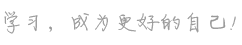## l是什么意思

1.英语字母中的第十二个字母
abbr.
1.=lake
2.=land
3.=late
4.=left
5.=length
6.=line
7.=lira
8.=leaf
9.=league
L.
abbr.
1.=Latin
2.=licentiate (in titles)
3.=Linnaean
4.=lodge (society)
5.=length

• a cgs unit of illumination equal to the brightness of a perfectly diffusing surface that emits or reflects one lumen per square centimeter
• the cardinal number that is the product of ten and five
• being ten more than forty
• the 12th letter of the Roman alphabet
• a metric unit of capacity equal to the volume of 1 kilogram of pure water at 4 degrees centigrade and 760 mm of mercury (or approximately 1.76 pints)

• L. L. L. 【医】 左[肺]下叶
• P and L abbr. =profit and loss 【商】盈亏,得失
• S & L abbr. =savings and loan association 中小金融联合会
• Apocynum L 【医】 茶叶花属
• L segregates 锭边偏析
• GAS & L abbr. =Great American Savings and Loan 美国大宗储蓄与贷款(银行)
• l shell l 层
• Arctium L 【医】 牛蒡属
• Areca L 【医】 槟榔属
• band l 【电】 L波段

• L n. 1.字母l
• l n. 1.英语字母中的第十二个字母 abbr. 1.=lake 2.=land 3.=late 4.=left 5.=length 6.=line 7.=lira 8.=l
• l/l 【缩写】
1.=line by line 一行一行的
2.=lower limit 下限
• R.L.L. 【医】 右下叶(肺)
• L.L.T. 伦敦起货条件；伦敦港岸上交货条件 见London landed terms.